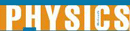# Fermions - Research Article from World of Physics

This encyclopedia article consists of approximately 3 pages of information about Fermions.
 This section contains 728 words (approx. 3 pages at 300 words per page) View a FREE sampleFermions and bosons are the two classes of elementary particles. Fermions are the particles that actually compose matter whereas bosons are associated with mediating the fundamental forces of nature between fermions. Fermions are particles whose quantum mechanical spin number is a half-integral (1/2, 3/2, 5/2, etc.) and obeys Fermi-Dirac statistics. In 1926 Paul Dirac and Werner Heisenberg applied the Pauli principle, which states that, the complete wave function, including both spatial and spin coordinates, of a system of identical fermions must be antisymmetric with respect to interchange of all of the coordinates of any two particles. This means that in a system of identical fermions each spin-orbit can hold no more than one fermion.

There are two classes of fermions, elementary fermions, such as leptons and quarks, and composite fermions, such as protons and neutrons. The leptons subclass is broken down into three groups or generations that each consist of one charged...

 This section contains 728 words (approx. 3 pages at 300 words per page) View a FREE sample